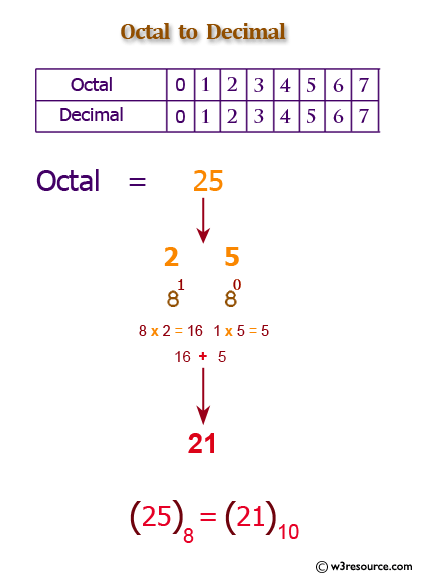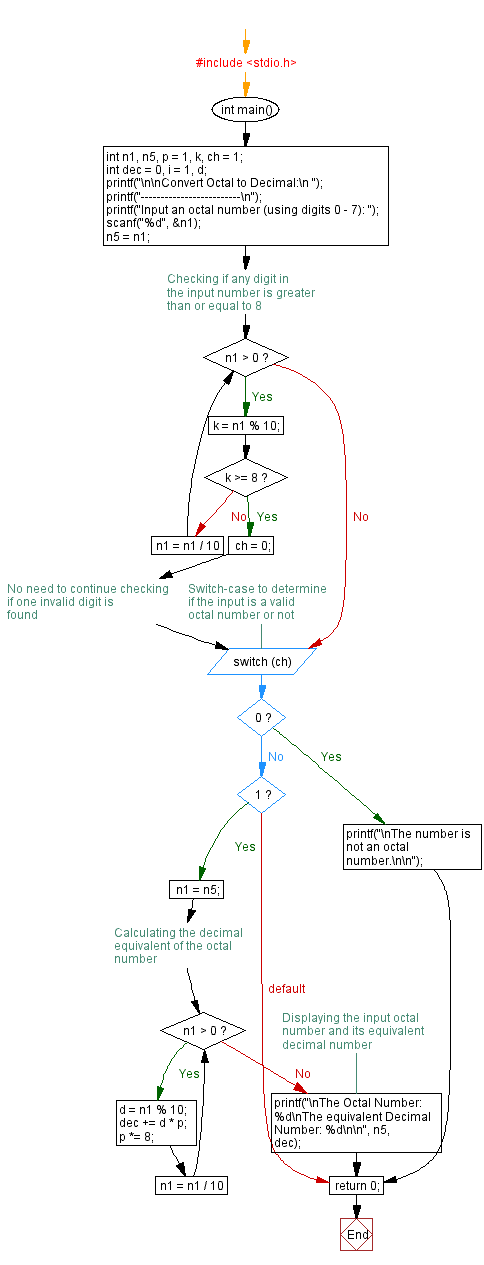﻿ C Program: Convert Octal number to a Decimal number - w3resource# C Exercises: Convert Octal number to a Decimal number

## C For Loop: Exercise-51 with Solution

Write a program in C to convert a Octal number to a Decimal number without using an array, function and while loop.

Pictorial Presentation:Sample Solution:

C Code:

``````#include <stdio.h>

void main()
{       int n1, n5,p=1,k,ch=1;
int dec=0,i=1,j,d;

printf("\n\nConvert Octal to Decimal:\n ");
printf("-------------------------\n");

printf("Input an octal number (using digit 0 - 7) :");
scanf("%d",&n1);
n5=n1;

for(;n1>0;n1=n1/10)
{
k=n1 % 10;
if(k>=8)
{
ch=0;
}
}

switch(ch)
{
case 0 :
printf("\nThe number is not an octal number. \n\n");
break;
case 1:
n1=n5;
for (j=n1;j>0;j=j/10)
{
d = j % 10;
if(i==1)
p=p*1;
else
p=p*8;

dec=dec+(d*p);
i++;
}
printf("\nThe Octal Number : %d\nThe equivalent Decimal  Number : %d \n\n",n5,dec);
break;
}
}
```
```

Sample Output:

```Convert Octal to Decimal:
-------------------------
Input an octal number (using digit 0 - 7) :745

The Octal Number : 745
The equivalent Decimal  Number : 485
```

Flowchart:C Programming Code Editor:

Improve this sample solution and post your code through Disqus.

What is the difficulty level of this exercise?

Test your Programming skills with w3resource's quiz.

﻿

## C Programming: Tips of the Day

You are seeing the ffffff because char is signed on your system. In C, vararg functions such as printf will promote all integers smaller than int to int. Since char is an integer (8-bit signed integer in your case), your chars are being promoted to int via sign-extension.

Since c0 and 80 have a leading 1-bit (and are negative as an 8-bit integer), they are being sign-extended while the others in your sample don't.

```char    int
c0 -> ffffffc0
80 -> ffffff80
61 -> 00000061
```
Here's a solution:
```char ch = 0xC0;
printf("%x", ch & 0xff);
```

This will mask out the upper bits and keep only the lower 8 bits that you want.

Ref : https://bit.ly/3vOLizM